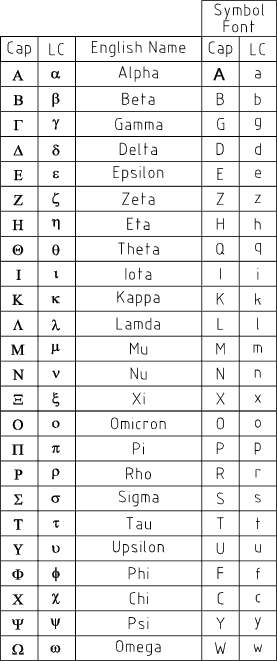Formulae_Index

Nomenclature

 Nomenclature Mathematics Dynamics Energy-Power Stress/Strain

 Symbol Description Units A Cross Section Area m2 a Acceleration m/sec2 D Diameter m 2 e Energy Joule (N.m) e Strain - E Young's Modulus N/m2 F Force N G Shear Modulus .. Modulus Of Rigity N/m2 I Second Moment of Area of a Section about its Neutral Axis m4 I Mass Moment of Inertia of a body about its axis of Rotation kg.m2 J Polar Moment of Inertia m4 J Mass Moment of Inertia of a body about its axis of Rotation kg.m2 m Mass kg M Moment N.m p Tensile/Compressive Stress N/m2 P Pressure N/m2 q Shear Stress N/m2 R Radius m s Distance m t Time sec t Thickness m T Torque N.m v Velocity m / s W Work Done Joule (N.m) W Beam Load N w Beam Distributed load N /m x Distance x direction m y Distance y direction m α Angular Acceleration s-2 ε Shear Strain Radian θ Angle Radian ν Poisson's Ratio - π Circumference/ Diameter 3.14159 (approx) σ Stress N / m2 τ Shear Stress N/m2 ω Angular Velocity sec-1

## Greek alphabet may be obtained with symbol FontFormulae_Index